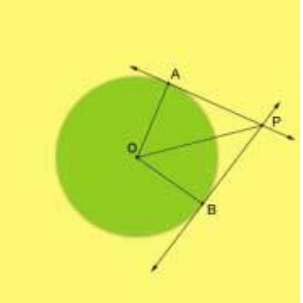Tangents drawn from an external point

## Objective:

To verify that the lengths of tangents drawn from an external point are equal.

## Theory:

### Related terms

• Circle – Set of all points in a plane that are equidistant from a given point called a center of the circle.
• Radius - The distance from the center to a point on the circle is called the radius of the circle.  Two circles are congruent if they have the same radius.

## Tangents to a circle:A tangent is a line in the plane of a circle that intersects the circle at exactly one point.

Tangent Lines to circles from the subject of several theorems, and play an important role in many geometrical constructions and proofs. Since the tangent line to a circle at any point P is perpendicular to the radius to that point.

It is observed that if two line segment from the same exterior point are tangent to the circle, then they are congruent. If AP and BP are tangent to a Circle O then AP = BP.